## Example Questions

← Previous 1 3 4 5 6 7 8

### Example Question #1 : Simplifying Expressions

If x + y = 4, what is the value of x + y – 6?

4

0

2

–2

6

–2

Explanation:

Substitute 4 for x + y in the expression given.

4 minus 6 equals –2.

### Example Question #2 : Simplifying Expressions

If 6 less than the product of 9 and a number is equal to 48, what is the number?

4

3

5

6

6

Explanation:

Write an equation for the written expression: 9x – 6 = 48.  When we solve for x we get x = 6.

### Example Question #3 : Simplifying Expressions

If  x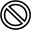y  = (5x - 4y)/y , find the value of y if 6y = 2.

2

4

10

5

5

Explanation:

If we substitute 6 in for x in the given equation and set our answer to 2, we can solve for y algebraically. 30 minus 4y divided by y equals 2 -->2y =30 -4y --> 6y =30 --> y=5.  We could also work from the answers and substitute each answer in and solve.

### Example Question #4 : Simplifying Expressions

Evaluate: (2x + 4)(x2 – 2x + 4)

2x3 + 16

2x3 – 4x2 + 8x

4x2 + 16x + 16

2x3 + 8x2 – 16x – 16

2x3 – 8x2 + 16x + 16

2x3 + 16

Explanation:

Multiply each term of the first factor by each term of the second factor and then combine like terms.

(2x + 4)(x2 – 2x + 4) = 2x3 – 4x2 + 8x  +  4x2 – 8x + 16 = 2x3 + 16

### Example Question #25 : Simplifying Expressions

Which of the following is equivalent to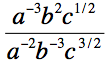?

ab/c

a2/(b5c)

b5/(ac)

abc

ab5c

b5/(ac)

Explanation:

First, we can use the property of exponents that xy/xz = xy–z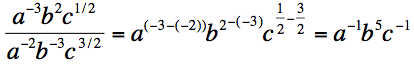Then we can use the property of exponents that states x–y = 1/xy

a–1b5c–1 = b5/ac

### Example Question #5 : Simplifying Expressions

Solve for x: 2y/3b = 5x/7a

15b/14ay

7ab/6y

14ay/15b

5by/3a

6ab/7y

14ay/15b

Explanation:

Cross multiply to get 14ay = 15bx, then divide by 15b to get x by itself.

### Example Question #6 : Simplifying Expressions

Three consecutive positive integers are added together. If the largest of the three numbers is m, find the sum of the three numbers in terms of m.

3m – 6

3m + 6

3m + 3

3m – 3

3m

3m – 3

Explanation:

Three consecutive positive integers are added together.  If the largest of the three numbers is m, find the sum of the three numbers in terms of m.

If m is the largest of three consecutive positive integers, then the integers must be:

m – 2, m – 1, and m, where m > 2.

The sum of these three numbers is:

m - 2 + m – 1 + m = 3m – 3

### Example Question #4 : How To Do Distance Problems

Sophie travels f miles in g hours.  She must drive another 30 miles at the same rate.  Find the total number of hours, in terms of f and g, that the trip will take.

g + f + 30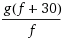g + f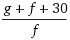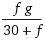Explanation:

Using d = rt, we know that first part of the trip can be represented by f = rg.  The second part of the trip can be represented by 30 = rx, where x is some unknown number of hours.  Note that the rate r is in both equations because Sophie is traveling at the same rate as mentioned in the problem.

Solve each equation for the time (g in equation 1, x in equation 2).

g = f/r

x = 30/r

The total time is the sum of these two times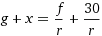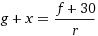Note that, from equation 1, r = f/g, so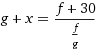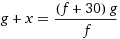=### Example Question #7 : Simplifying Expressions

If ab = 10 and bc = 15, then what is the value of (c – a)/(a + 2b + c)?

1/5

5

2/3

150

3/2

1/5

Explanation:

a + b = 10

b + c = 15

------------

a + b + b + c = 10 + 15

a + 2b + c = 25 (this is the denominator of the answer)

Subtract the two equations:

b + c = 15

a + b = 10

------------

b + c – (a + b) = 15 – 10

c – a = 5 (this is the numerator of the answer)

5/25 = 1/5

### Example Question #8 : Simplifying Expressions

If a = 2b, 3b = c, and 2c = 3d, what is the value of d/a?

3

1

2/3

3/2

2

1

Explanation:

Eq 1: a = 2b

Eq 2: 3b = c

Eq 3: 2c = 3d

Rewrite Eq. 3 substituting using Eq. 2.

2(3b) = 3d (because c = 3b)

6b = 3d (simplify)

2b = d (divide by 3)

Since a and d both equal 2b, a = d.  Therefore, d/a = 1.

← Previous 1 3 4 5 6 7 8